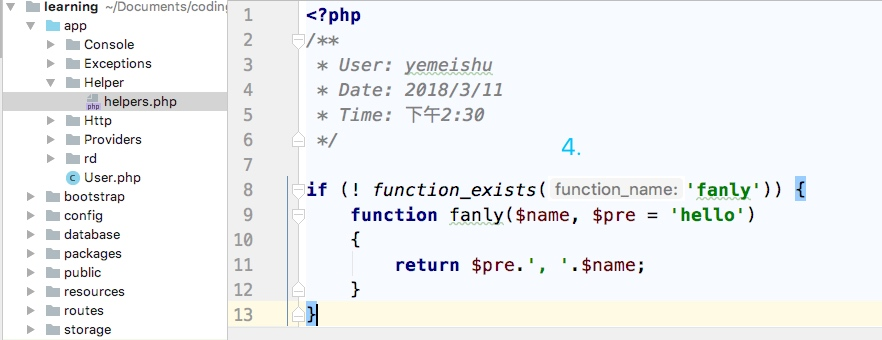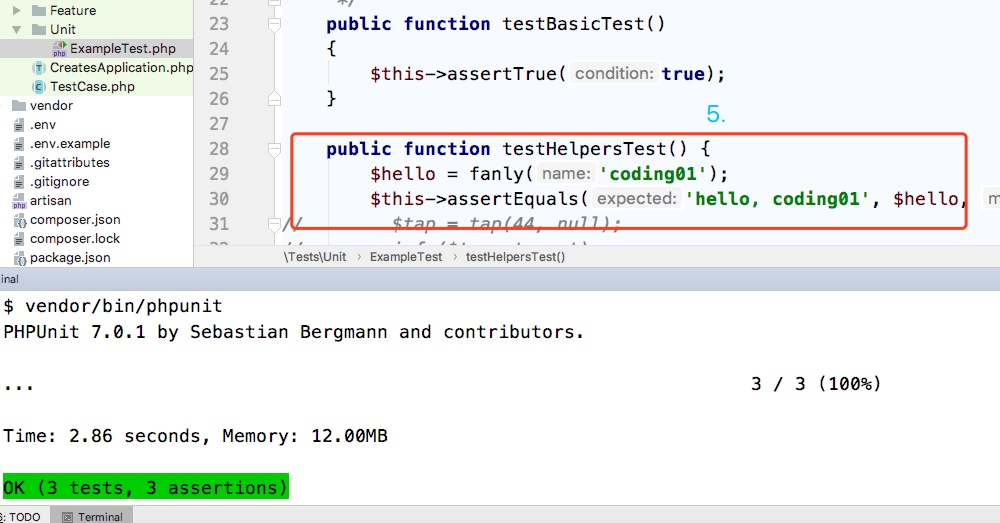# 玩转 Laravel Helpers

## 4 个好玩的 Helpers 函数

### data_get()

The data_get function retrieves a value from a nested array or object using "dot" notation:

The data_get function also accepts a default value, which will be returned if the specified key is not found

data_get() 函数利用“.”符号，从嵌套数组或者对象中检索数值，其中第三个参数是设置默认值，当检索健不存在时。

``````\$array = ['albums' => ['rock' => ['count' => 75]]];

\$count = data_get(\$array, 'albums.rock.count');
// 75

\$avgCost = data_get(\$array, 'albums.rock.avg_cost', 0);
// 0

\$object->albums->rock->count = 75;

\$count = data_get(\$object, 'albums.rock.count');
// 75

\$avgCost = data_get(\$object, 'albums.rock.avg_cost', 0);
// 0
``````

``````\$array = ['albums' => ['rock' => ['count' => 75], 'punk' => ['count' => 12]]];

\$counts = data_get(\$array, 'albums.*.count');
// [75, 12]

\$array = ['albums' => ['rock', 'punk' => ['count' => 12]]];

\$counts = data_get(\$array, 'albums.*.count');
// [NULL, 12]
``````

### str_plural()

``````str_plural('dog'); // dogs
str_plural('cat'); // cats

str_plural('dog', 2); // dogs
str_plural('cat', 1); // cat

str_plural('child'); // children
str_plural('person'); // people
str_plural('fish'); // fish
str_plural('deer', 2); // deer

str_singular('children') // child
``````

### value() and with()

value() 函数根据传入的值，返回数据，如果，传入的是闭包函数，则会直接运行闭包函数，返回结果：

``````\$result = value(5);
// 5

\$result = value(function () {
return false;
});
// false
``````

value() 个人觉得，更多用在于高阶函数中，闭包函数作为高阶函数的传值传入；

``````\$callback = function (\$value) {
return (is_numeric(\$value)) ? \$value * 2 : 0;
};

\$result = with(5, \$callback);

// 10

\$result = with(null, \$callback);

// 0

\$result = with(5, null);

// 5
``````

### tap()

``````<?php
function tap(\$value, \$callback)
{
\$callback(\$value);
return \$value;
}
``````

``````<?php
return tap(\$user)->update([
'name' => \$name,
'age' => \$age,
]);
``````

### 其它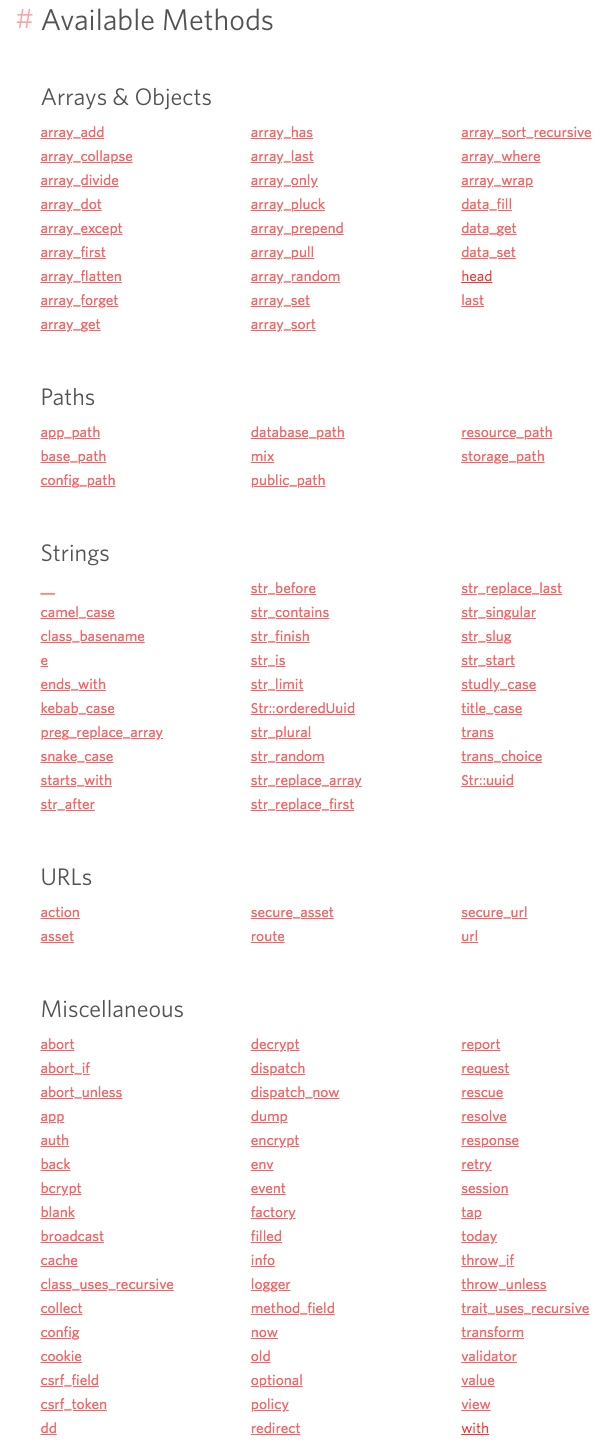## Helpers 函数实现原理

``````"src/Illuminate/Foundation/helpers.php",

"src/Illuminate/Support/helpers.php"
``````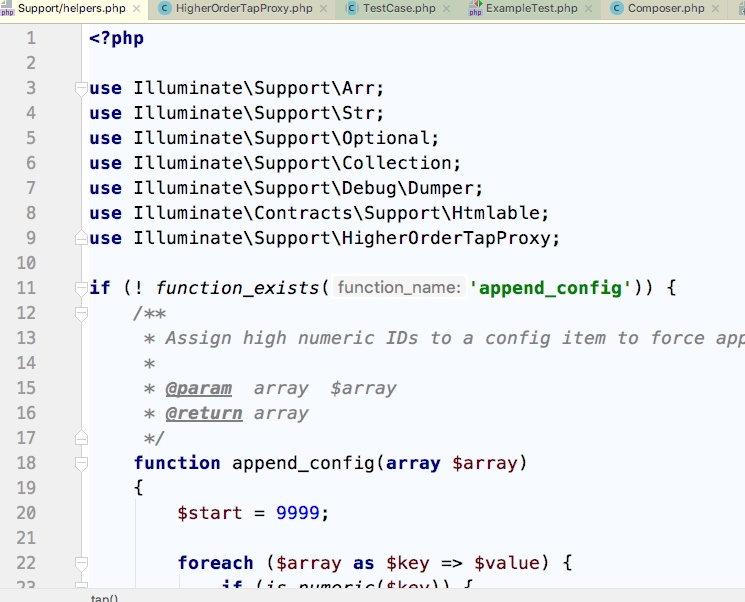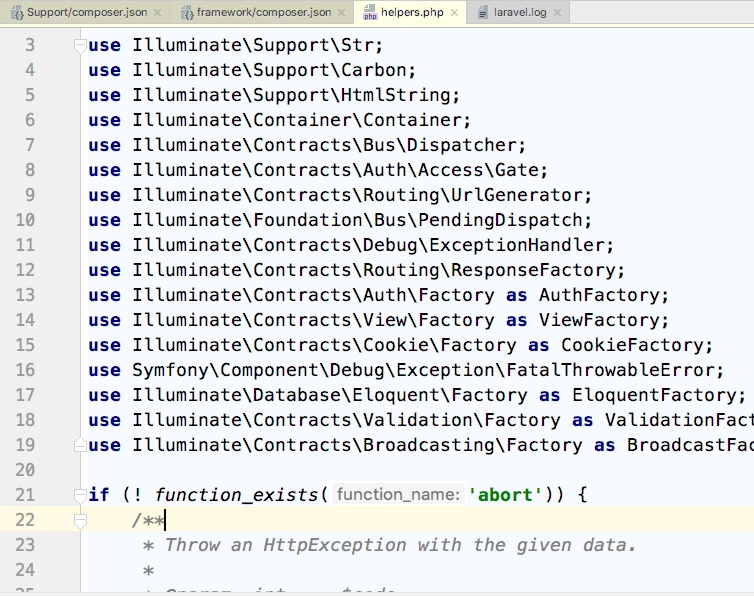Files

``````{
"files": ["src/MyLibrary/functions.php"]
}
}
``````

``````if (! function_exists('tap')) {
/**
* Call the given Closure with the given value then return the value.
*
* @param  mixed  \$value
* @param  callable|null  \$callback
* @return mixed
*/
function tap(\$value, \$callback = null)
{
if (is_null(\$callback)) {
return new HigherOrderTapProxy(\$value);
}

\$callback(\$value);

return \$value;
}
}
``````

``````if (! function_exists('xxx')) {
function xxx() {
// ...
}
}
``````

## 自定义 Helpers 函数

1. 在项目中创建 helper.php 文件
2. 在 composer.json 中将 helper 文件 autoload 载入
4. 在 helper.php 文件写入我们的全局函数
5. test